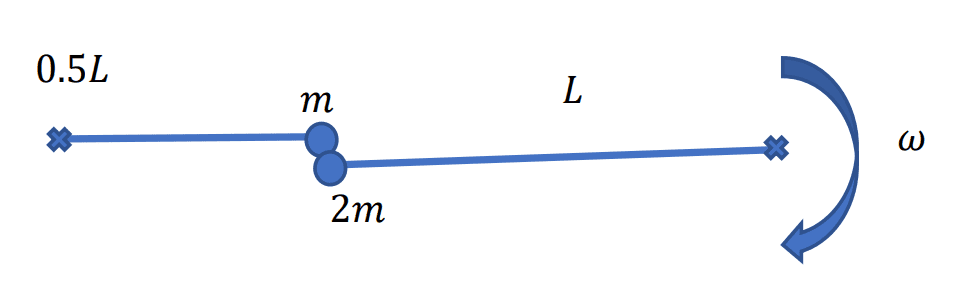# Calculating the energy lost during a collision + conservation of angular momentum

Combinatorics
Homework Statement:
A point mass "2m" is rotating around a fixed axis and collides with a stationary mass "m" that starts rotating around another fixed axis. $$\omega$$ is the angular velocity of mass $$2m$$ before the collision and $$0.8\omega$$ is its velocity after the collision.
I need to calculate the energy lost during the collision.
Relevant Equations:
J=mvr
J initial = J final?
E_k = 0.5mv^2
Unfortunately, I couldn't arrive to the correct answer ($$=0.28mL^2 \omega^2$$ ) and will be happy to understand what am I doing wrong.

**My attempt:**

Using $$E_k = \frac{1}{2} I \omega^2$$
I obtain that the difference I need to calculate is
$$\frac{1}{2} (2mL^2)(0.8\omega)^2 + \frac{1}{2} \left( m \frac{1}{2}^2 L^2 \right) \omega_2 ^2 - \frac{1}{2} (2mL^2) \omega^2,$$
where $$\omega_2$$ is the angular velocity of mass $$m$$ after the collision. To calculate its velocity, I use conservation of angular momentum and the formula $$J=mvr$$ for a point mass rotating around a fixed axis:
$$J_i = -2m\omega L^2 = J_f = -2m 0.8 \omega L^2 + m\omega_2 \frac{1}{2}L^2 \Leftrightarrow \omega_2 = -\frac{36}{5}\omega.$$
And therefore the difference is $$6.12mL^2 \omega^2$$.Last edited:

Homework Helper
Gold Member
2022 Award
That's hard to read. You need two dollar signs (or two hash signs) to delimit your Latex.

What's the question or quantity you're trying to find?

•Leo Liu
Combinatorics
That's hard to read. You need two dollar signs (or two hash signs) to delimit your Latex.

What's the question or quantity you're trying to find?
I apologize for not noticing the equations didn't appear as I wanted. I have now edited the thread.

Homework Helper
Gold Member
2022 Award
I apologize for not noticing the equations didn't appear as I wanted. I have now edited the thread.
How are you using conservation of angular momentum here? About which point is angular momentum conserved?

Combinatorics
How are you using conservation of angular momentum here? About which point is angular momentum conserved?
This is probably what I didn't understand. I used total angular momentum wrt the two fixed axes, since I couldn't think of any other way to calculate the angular velocity $$\omega_2 of the second point mass. How can I solve this question in another way? Science Advisor Homework Helper Gold Member 2022 Award This is probably what I didn't understand. I used total angular momentum wrt the two fixed axes, since I couldn't think of any other way to calculate the angular velocity$$\omega_2\$ of the second point mass. How can I solve this question in another way?
Angular momentum is always measured relative to some point or axis, so you can't mix and match your axes like that.

Perhaps there's a simple solution. Focus on the collision itself.

Combinatorics
Angular momentum is always measured relative to some point or axis, so you can't mix and match your axes like that.

Perhaps there's a simple solution. Focus on the collision itself.
Is it true that close enough to the collision I can just use conservation of linear momentum to find the velocity of the second mass after the collision?

Homework Helper
Gold Member
2022 Award
Is it true that close enough to the collision I can just use conservation of linear momentum to find the velocity of the second mass after the collision?
You could always try and see what answer you get.

Combinatorics
You could always try and see what answer you get.
Using conservation of linear momentum, I obtain that $$\omega_2 = 0.2\omega$$. Substituting in the energy difference gives $$-0.355 mL^2 \omega^2$$, which is still not the right answer... What am I doing wrong here?

Thank you

Homework Helper
Gold Member
2022 Award
Using conservation of linear momentum, I obtain that $$\omega_2 = 0.2\omega$$. Substituting in the energy difference gives $$-0.355 mL^2 \omega^2$$, which is still not the right answer... What am I doing wrong here?

Thank you
You got the wrong answer. I can't tell what you're doing wrong without seeing your calculations.

Homework Helper
Gold Member
2022 Award
Using conservation of linear momentum, I obtain that $$\omega_2 = 0.2\omega$$.

•Combinatorics
Combinatorics
You got the wrong answer. I can't tell what you're doing wrong without seeing your calculations.
Thanks for your feedback! here is my attempt. Using conservation of linear momentum, I obtain
$$2m\cdot \frac{\omega}{L} = m\cdot \frac{\omega_2}{0.5L} + 2m\cdot \frac{0.8\omega}{L}$$
where I used $$v=\frac{\omega}{L}$$.
Solving this equation implies
$$\omega_2 = 0.2\omega.$$
When I substitute this expression into the energy difference:
$$\frac{1}{2} (2mL^2)(0.8\omega)^2 + \frac{1}{2} \left( m \frac{1}{2}^2 L^2 \right) \omega_2 ^2 - \frac{1}{2} (2mL^2) \omega^2,$$
I obtain
$$-0.355 mL^2 \omega^2.$$

What am I doing wrong?

Thank you very much again!

Homework Helper
Gold Member
2022 Award
The speed of the mass is not ##\omega /L##.

•Combinatorics
Combinatorics
The speed of the mass is not ##\omega /L##.
Thank you PeroK, you helped a lot!

•